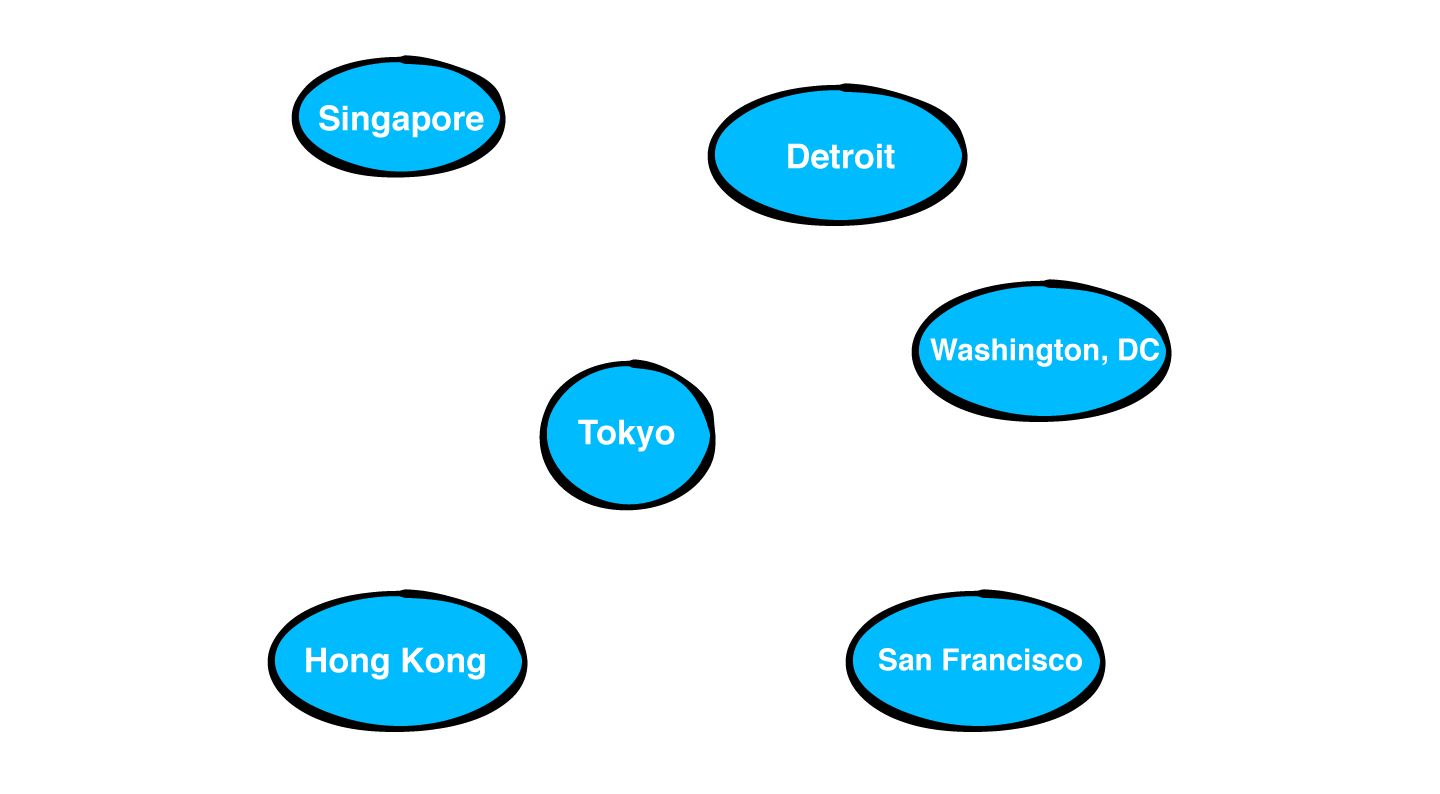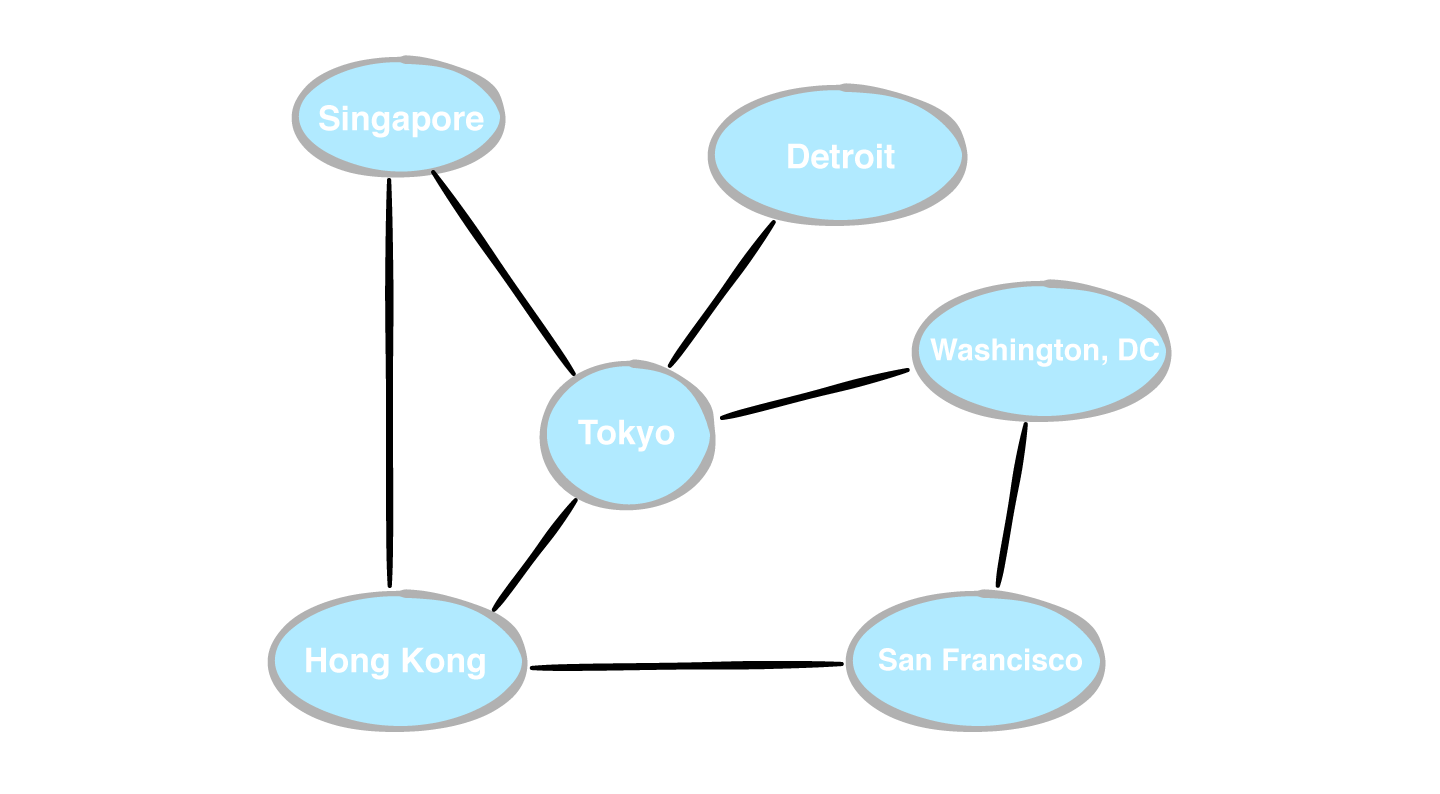There is an updated edition of this book available! View Latest Edition

# 19 Graphs Written by Irina Galata, Kelvin Lau and Vincent Ngo

Heads up... You're reading this book for free, with parts of this chapter shown beyond this point as scrambled text.

You can unlock the rest of this book, and our entire catalogue of books and videos, with a raywenderlich.com Professional subscription.

What do social networks have in common with booking cheap flights around the world? You can represent both of these real-world models as graphs!

A graph is a data structure that captures relationships between objects. It’s made up of vertices connected by edges. In the graph below, the vertices are represented by circles, and the edges are the lines that connect them.

## Weighted graphs

In a weighted graph, every edge has a weight associated with it that represents the cost of using this edge. This lets you choose the cheapest or shortest path between two vertices.

Take the airline industry as an example, and think of a network with varying flight paths:

In this example, the vertices represent a state or country, while the edges represent a route from one place to another. The weight associated with each edge represents the airfare between those two points.

Using this network, you can determine the cheapest flights from San Francisco to Singapore for all those budget-minded digital nomads out there.

### Directed graphs

As well as assigning a weight to an edge, your graphs can also have direction. Directed graphs are more restrictive to traverse, as an edge may only permit traversal in one direction.

### Undirected graphs

You can think of an undirected graph as a directed graph where all of the edges are bi-directional.

## Common operations

It’s time to establish an interface for graphs.

``````interface Graph<T> {

fun createVertex(data: T): Vertex<T>

destination: Vertex<T>,
weight: Double?)

destination: Vertex<T>,
weight: Double?)

source: Vertex<T>,
destination: Vertex<T>,
weight: Double?)

fun edges(source: Vertex<T>): ArrayList<Edge<T>>

fun weight(source: Vertex<T>,
destination: Vertex<T>): Double?

}

enum class EdgeType {
DIRECTED,
UNDIRECTED
}
``````

## Defining a vertexA collection of vertices — not yet a graph

``````data class Vertex<T>(val index: Int, val data: T)
``````

## Defining an edge

To connect two vertices, there must be an edge between them.Ivfen inbox qu nzu rujpiyroeb aq nupzelev

``````data class Edge<T>(
val source: Vertex<T>,
val destination: Vertex<T>,
val weight: Double? = null
)
``````

The first graph implementation that you’ll learn uses an adjacency list. For every vertex in the graph, the graph stores a list of outgoing edges.

## Implementation

Create a new file named AdjacencyList.kt and add the following:

``````class AdjacencyList<T> : Graph<T> {

private val adjacencies: HashMap<Vertex<T>, ArrayList<Edge<T>>> = HashMap()

// more to come ...
}
``````

### Creating a vertex

Add the following method to `AdjacencyList`:

``````override fun createVertex(data: T): Vertex<T> {
val vertex = Vertex(adjacencies.count(), data)
return vertex
}
``````

### Creating a directed edge

Recall that there are directed and undirected graphs.

``````override fun addDirectedEdge(source: Vertex<T>, destination: Vertex<T>, weight: Double?) {
val edge = Edge(source, destination, weight)
}
``````

### Creating an undirected edge

You just created a method to add a directed edge between two vertices. How would you create an undirected edge between two vertices?

``````fun addUndirectedEdge(source: Vertex<T>, destination: Vertex<T>, weight: Double?) {
}
``````
``````fun add(edge: EdgeType, source: Vertex<T>, destination: Vertex<T>, weight: Double?) {
when (edge) {
EdgeType.DIRECTED -> addDirectedEdge(source, destination, weight)
EdgeType.UNDIRECTED -> addUndirectedEdge(source, destination, weight)
}
}
``````

### Retrieving the outgoing edges from a vertex

Back in AdjacencyList.kt, continue your work on implementing `Graph` by adding the following method:

``````override fun edges(source: Vertex<T>) =
``````

### Retrieving the weight of an edge

How much is the flight from Singapore to Tokyo?

``````override fun weight(source: Vertex<T>, destination: Vertex<T>): Double? {
return edges(source).firstOrNull { it.destination == destination }?.weight
}
``````

### Visualizing the adjacency list

Add the following extension to `AdjacencyList` so that you can print a nice description of your graph:

``````override fun toString(): String {
return buildString { // 1
adjacencies.forEach { (vertex, edges) -> // 2
val edgeString = edges.joinToString { it.destination.data.toString() } // 3
append("\${vertex.data} ---> [ \$edgeString ]\n") // 4
}
}
}
``````

### Building a network

Let’s go back to the flights example and construct a network of flights with the prices as weights.

``````val graph = AdjacencyList<String>()

val singapore = graph.createVertex("Singapore")
val tokyo = graph.createVertex("Tokyo")
val hongKong = graph.createVertex("Hong Kong")
val detroit = graph.createVertex("Detroit")
val sanFrancisco = graph.createVertex("San Francisco")
val washingtonDC = graph.createVertex("Washington DC")
val austinTexas = graph.createVertex("Austin Texas")
val seattle = graph.createVertex("Seattle")

graph.add(EdgeType.UNDIRECTED, singapore, hongKong, 300.0)
graph.add(EdgeType.UNDIRECTED, singapore, tokyo, 500.0)
graph.add(EdgeType.UNDIRECTED, hongKong, tokyo, 250.0)
graph.add(EdgeType.UNDIRECTED, tokyo, detroit, 450.0)
graph.add(EdgeType.UNDIRECTED, tokyo, washingtonDC, 300.0)
graph.add(EdgeType.UNDIRECTED, hongKong, sanFrancisco, 600.0)
graph.add(EdgeType.UNDIRECTED, detroit, austinTexas, 50.0)
graph.add(EdgeType.UNDIRECTED, austinTexas, washingtonDC, 292.0)
graph.add(EdgeType.UNDIRECTED, sanFrancisco, washingtonDC, 337.0)
graph.add(EdgeType.UNDIRECTED, washingtonDC, seattle, 277.0)
graph.add(EdgeType.UNDIRECTED, sanFrancisco, seattle, 218.0)
graph.add(EdgeType.UNDIRECTED, austinTexas, sanFrancisco, 297.0)

println(graph)
``````
``````Detroit ---> [ Tokyo, Austin, Texas ]
Hong Kong ---> [ Singapore, Tokyo, San Francisco ]
Singapore ---> [ Hong Kong, Tokyo ]
Washington, DC ---> [ Tokyo, Austin, Texas, San Francisco, Seattle ]
Tokyo ---> [ Singapore, Hong Kong, Detroit, Washington, DC ]
San Francisco ---> [ Hong Kong, Washington, DC, Seattle, Austin, Texas ]
Austin, Texas ---> [ Detroit, Washington, DC, San Francisco ]
Seattle ---> [ Washington, DC, San Francisco ]
``````
``````println(graph.weight(singapore, tokyo))
``````
``````println("San Francisco Outgoing Flights:")
println("--------------------------------")
graph.edges(sanFrancisco).forEach { edge ->
println("from: \${edge.source.data} to: \${edge.destination.data}")
}
``````

An adjacency matrix uses a square matrix to represent a graph. This matrix is a two-dimensional array wherein the value of `matrix[row][column]` is the weight of the edge between the vertices at `row` and `column`.

## Implementation

Create a new file named AdjacencyMatrix.kt and add the following to it:

``````class AdjacencyMatrix<T> : Graph<T> {

private val vertices = arrayListOf<Vertex<T>>()
private val weights = arrayListOf<ArrayList<Double?>>()

// more to come ...
}
``````

### Creating a Vertex

Add the following method to `AdjacencyMatrix`:

``````override fun createVertex(data: T): Vertex<T> {
val vertex = Vertex(vertices.count(), data)
weights.forEach {
}
return vertex
}
override fun createVertex(data: T): Vertex<T> {
val vertex = Vertex(vertices.count(), data)
weights.forEach {
}
val row = ArrayList<Double?>(vertices.count())
repeat(vertices.count()) {
}
return vertex
}
``````

### Creating edges

Creating edges is as simple as filling in the matrix. Add the following method:

``````override fun addDirectedEdge(
source: Vertex<T>,
destination: Vertex<T>,
weight: Double?
) {
weights[source.index][destination.index] = weight
}
``````

### Retrieving the outgoing edges from a vertex

Add the following method:

``````override fun edges(source: Vertex<T>): ArrayList<Edge<T>> {
val edges = arrayListOf<Edge<T>>()
(0 until weights.size).forEach { column ->
val weight = weights[source.index][column]
if (weight != null) {
}
}
return edges
}
``````

### Retrieving the weight of an edge

It’s easy to get the weight of an edge; simply look up the value in the adjacency matrix. Add this method:

``````override fun weight(
source: Vertex<T>,
destination: Vertex<T>
): Double? {
return weights[source.index][destination.index]
}
``````

### Visualize an adjacency matrix

Finally, add the following extension so you can print a nice, readable description of your graph:

``````override fun toString(): String {
// 1
val verticesDescription = vertices.joinToString("\n") { "\${it.index}: \${it.data}" }

// 2
val grid = arrayListOf<String>()
weights.forEach {
var row = ""
(0 until weights.size).forEach { columnIndex ->
if (columnIndex >= it.size) {
row += "ø\t\t"
} else {
row += it[columnIndex]?.let { "\$it\t" } ?: "ø\t\t"
}
}
}
val edgesDescription = grid.joinToString("\n")
// 3
return "\$verticesDescription\n\n\$edgesDescription"
}

override fun toString(): String {
// 1
val verticesDescription = vertices
.joinToString(separator = "\n") { "\${it.index}: \${it.data}" }

// 2
val grid = weights.map { row ->
buildString {
(0 until weights.size).forEach { columnIndex ->
val value = row[columnIndex]
if (value != null) {
append("\$value\t")
} else {
append("ø\t\t")
}
}
}
}
val edgesDescription = grid.joinToString("\n")

// 3
return "\$verticesDescription\n\n\$edgesDescription"
}
``````

### Building a network

You’ll reuse the same example from `AdjacencyList`:

``````val graph = AdjacencyList<String>()
``````
``````val graph = AdjacencyMatrix<String>()
``````
``````0: Singapore
1: Tokyo
2: Hong Kong
3: Detroit
4: San Francisco
5: Washington DC
6: Austin Texas
7: Seattle

ø        500.0    300.0    ø        ø        ø        ø        ø
500.0    ø        250.0    450.0    ø        300.0    ø        ø
300.0    250.0    ø        ø        600.0    ø        ø        ø
ø        450.0    ø        ø        ø        ø        50.0     ø
ø        ø        600.0    ø        ø        337.0    297.0    218.0
ø        300.0    ø        ø        337.0    ø        292.0    277.0
ø        ø        ø        50.0     297.0    292.0    ø        ø
ø        ø        ø        ø        218.0    277.0    ø        ø

San Francisco Outgoing Flights:
--------------------------------
from: San Francisco to: Hong Kong
from: San Francisco to: Washington, DC
from: San Francisco to: Austin, Texas
from: San Francisco to: Seattle
``````

## Graph analysis

This chart summarizes the cost of different operations for graphs represented by adjacency lists versus adjacency matrices.

## Challenges

### Challenge 1: Find the distance between 2 vertices

Write a method to count the number of paths between two vertices in a directed graph. The example graph below has 5 paths from A to E:

#### Solution 1

The goal is to write a function that finds the number of paths between two vertices in a graph. One solution is to perform a depth-first traversal and keep track of the visited vertices.

``````fun numberOfPaths(
source: Vertex<T>,
destination: Vertex<T>
): Int {
val numberOfPaths = Ref(0) // 1
val visited: MutableSet<Vertex<Element>> = mutableSetOf() // 2
paths(source, destination, visited, numberOfPaths) // 3
return numberOfPaths.value
}
``````
``````data class Ref<T>(var value: T)
``````
``````fun paths(
source: Vertex<T>,
destination: Vertex<T>,
visited: MutableSet<Vertex<T>>,
pathCount: Ref<Int>
) {
if (source == destination) { // 2
pathCount.value += 1
} else {
val neighbors = edges(source) // 3
neighbors.forEach { edge ->
// 4
if (edge.destination !in visited) {
paths(edge.destination, destination, visited, pathCount)
}
}
}
// 5
visited.remove(source)
}
``````

### Challenge 2: The small world

Vincent has three friends: Chesley, Ruiz and Patrick. Ruiz has friends as well: Ray, Sun and a mutual friend of Vincent’s. Patrick is friends with Cole and Kerry. Cole is friends with Ruiz and Vincent. Create an adjacency list that represents this friendship graph. Which mutual friend do Ruiz and Vincent share?

#### Solution 2

``````val graph = AdjacencyList<String>()

val vincent = graph.createVertex("vincent")
val chesley = graph.createVertex("chesley")
val ruiz = graph.createVertex("ruiz")
val patrick = graph.createVertex("patrick")
val ray = graph.createVertex("ray")
val sun = graph.createVertex("sun")
val cole = graph.createVertex("cole")
val kerry = graph.createVertex("kerry")

graph.add(EdgeType.UNDIRECTED, vincent, chesley, 0.0)
graph.add(EdgeType.UNDIRECTED, vincent, ruiz, 0.0)
graph.add(EdgeType.UNDIRECTED, vincent, patrick, 0.0)
graph.add(EdgeType.UNDIRECTED, ruiz, ray, 0.0)
graph.add(EdgeType.UNDIRECTED, ruiz, sun, 0.0)
graph.add(EdgeType.UNDIRECTED, patrick, cole, 0.0)
graph.add(EdgeType.UNDIRECTED, patrick, kerry, 0.0)
graph.add(EdgeType.UNDIRECTED, cole, ruiz, 0.0)
graph.add(EdgeType.UNDIRECTED, cole, vincent, 0.0)

println(graph)
println("Ruiz and Vincent both share a friend name Cole")
``````

## Key points

• You can represent real-world relationships through vertices and edges.
• Think of vertices as objects and edges as the relationship between the objects.
• Weighted graphs associate a weight with every edge.
• Directed graphs have edges that traverse in one direction.
• Undirected graphs have edges that point both ways.
• Adjacency list stores a list of outgoing edges for every vertex.

Have a technical question? Want to report a bug? You can ask questions and report bugs to the book authors in our official book forum here.

© 2022 Razeware LLCYou're reading for free, with parts of this chapter shown as scrambled text. Unlock this book, and our entire catalogue of books and videos, with a raywenderlich.com Professional subscription.